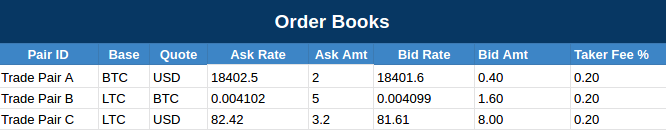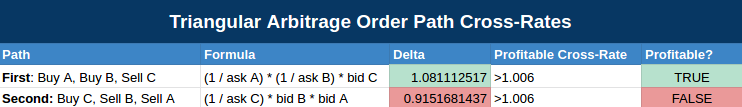# Cryptocurrency triangular arbitrage: How does it work?

Triangular arbitrage is a commonly known technique for exploiting price differences between assets to try and make a quick and low-risk profit. If you’ve been wondering how triangular arbitrage works and how it applies to Bitcoin, Ethereum and other cryptocurrencies, you’re in luck! In this post I’ll explain the basics of cryptocurrency triangular arbitrage, how to find triangular arbitrage opportunities, and some of the basic math behind calculating profits and orders.

(P.S. If you want a fully automated triangular arbitrage bot, we’ve built a powerful and secure platform that you can try for free! You can learn more in our blog post Master crypto triangular arbitrage with Coygo’s new trading bot).*

Triangular arbitrage is a form of intra-exchange arbitrage, meaning it’s performed on one exchange. If you’re interested in learning about inter-exchange arbitrage that purchase cryptocurrencies on one exchange and sells on another, please see our other blog post: How to Profit From Crypto Arbitrage Trading.## What is triangular arbitrage?

Triangular arbitrage involves trading between three different assets and exploiting price differences to try and make a profit. For example, if you have BTC you may buy ETH with BTC, then buy LTC with that ETH, then finally sell that LTC back to BTC. If the bid and ask rates of each trade pair (ETH-BTC, LTC-ETH and LTC-BTC) are right, there can be opportunity for a profit.

## How can I find crypto triangular arbitrage opportunities?

To find opportunities that are profitable you can do a bit of math to determine if a cross-rate is overvalued, meaning that there is a price discrepancy when trading between three different assets.

I will show by example, let’s define the following values:

• Trade pair A — BTC-USD

• Trade pair B — LTC-BTC

• Trade pair C — LTC-USD

• Trading fees — In this example I’ll assume that each trade pair has a 0.2% taker fee.

We will use the following order book data for this example, which shows the ask and bid for each trade pair:Example order book data with ask and bid rates and amounts

There are two different paths we can use to trade:

2. Buy on “Trade pair B”. We now have BTC, and we buy LTC with BTC on LTC-BTC.

3. Sell on “Trade pair C”. We now have LTC, and we sell LTC for USD on LTC-USD, ending with a balance of USD again.

The formula to calculate the cross-rate formula for the First path is:

If this cross-rate is > 1, it’s overvalued. If it’s > the sum of each trade pair’s trading fees it would be profitable. In this example, our trading fees are 0.2% on each pair so the cross-rate must be > 1.006. (0.2% is 0.002, so it’s 1 + 0.002 + 0.002 + 0.002).

Second path — Buy, Sell, Sell

2. Sell on “Trade pair B”. We now have LTC, and we sell LTC for BTC on LTC-BTC.

3. Sell on “Trade pair A”. We now have BTC, and we sell BTC for USD on BTC-USD, ending with a balance of USD again.

The formula to calculate the cross-rate formula for the Second path is:

If this cross-rate is > 1, it’s overvalued. If it’s > the sum of each trade pair’s trading fees it would be profitable. In this example, our trading fees are 0.2% on each pair so the cross-rate must be > 1.006. (0.2% is 0.002, so it’s 1 + 0.002 + 0.002 + 0.002).

### Finding the profitable order path

Using these formulas above, we can calculate the cross-rate of each path and we will get the following results which shows that the First path is profitable after trading fees.Calculations of each order path’s cross-rate and profit

### Accounting for slippage

We must also calculate the correct order amounts to avoid slippage. For example the first ask that you’ll be filling may only be for 0.5 BTC, so if you submit an order larger than that you’ll end up incurring slippage and not get the rate that you expected. This is a complex topic that I won’t be going into too deeply in this post, but basically it’s easiest to do the math for each trade pair’s ask and/or bid that you’ll be filling to figure out how much it’s value is in the Starting asset. For example, if the LTC-BTC ask you’re filling is 1.5 LTC you can figure out how much BTC that 1.5 LTC is equivalent to. Do this with each trade pair, and then find the smallest order size among the three orders and use that as your maximum order size since that’s the largest order you can submit without slippage.